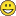# New to Qlik Sense

Discussion board where members can get started with Qlik Sense.

New Contributor II

## Sum Sales by month

Hello,

I'm using QlikSens Desktop

I want to create KPI visualisation whith show total SALES for the current month.

I Created a variable currentMonth = Month(today())

currentMonth value = juil.

currentMonthTotalSales = Sum({< [DateCmd_PK-Date_PK.autoCalendar.Month]  = {'juil.'}> } Total_HT)  ---> WORKS

currentMonthTotalSales = Sum({< [DateCmd_PK-Date_PK.autoCalendar.Month]  = {currentMonth}> } Total_HT)  DOES NOT WORKS

I also Tried

currentMonthTotalSales = Sum({< [DateCmd_PK-Date_PK.autoCalendar.MonthRelNo]  = {6}> } Total_HT)  --> this does not work also

could some one help me on this issue please ?

Tags (3)
7 Replies
Esteemed Contributor III

## Re: Sum Sales by month

try this

= Sum({< [DateCmd_PK-Date_PK.autoCalendar.Month]  = {"\$(=MaxString(YourMonthFiled))"}> } Total_HT)Partner

## Re: Sum Sales by month

currentMonthTotalSales = Sum({< [DateCmd_PK-Date_PK.autoCalendar.Month]  = {\${currentMonth}}> } Total_HT)

Does above work?

Valued Contributor

## Re: Sum Sales by month

month() returns a dual value and is likely using the number for comparison, try using monthname()

currentMonth = MonthName(today())

Honored Contributor

## Re: Sum Sales by month

currentMonth = Month(Max(date));

Expression:

currentMonthTotalSales = Sum({< [DateCmd_PK-Date_PK.autoCalendar.Month]  = {'\$( =\$(currentMonth) )'}> } Total_HT)

Valued Contributor III

## Re: Sum Sales by month

currentMonthTotalSales = Sum({< [DateCmd_PK-Date_PK.autoCalendar.Month]  ={\$(=currentMonth)}> } Total_HT) //with parentesis...

Or:

currentMonthTotalSales = Sum({< [DateCmd_PK-Date_PK.autoCalendar.Month]={\$(=Max( [DateCmd_PK-Date_PK.autoCalendar.Month]))}> } Total_HT)

Or

currentMonthTotalSales = Sum({< [DateCmd_PK-Date_PK.autoCalendar.Month]={\$(=Month (today ()))}> } Total_HT)

Thiago Justen Teixeira Gonçalves
WhatsApp: 24 98152-1675
Skype: justen.thiago
New Contributor II

## Re: Sum Sales by month

I think that all your answer where rightI m new to QlikSens and the syntax wasn't pretty easy to understand for retriving local variable for me !!
Special thanks to "Thiago Justen Teixeira"
it's all good !!

Valued Contributor III